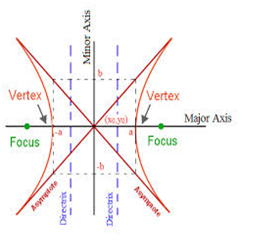Courses

# Notes | EduRev

## JEE : Notes | EduRev

The document Notes | EduRev is a part of the JEE Course Mock Test Series for JEE Main & Advanced 2021.
All you need of JEE at this link: JEE
• The standard equation of hyperbola with reference to its principal axis along the coordinate axis is given by x2/a2 - y2/b2 = 1, where b2 = a2 (e2 -1)
• The foci of the hyperbola are S(ae, 0) and S’ = (-ae, 0)
• Equations of the directrices are given by x = a/e and x = -a/e
• The coordinates of vertices are A’ = (-a, 0) and A = (a,0)
• The length of latus rectum is 2b2/a = 2a(e2 - 1)
• The length of the transverse axis of the hyperbola is 2a.
• The difference of the focal distances of any point on the hyperbola is constant and is equal to transverse axis.
• x2/a- y2/b2 = 1 and -x2/a2 + y2/b2 = 1 are conjugate hyperbola of each other.
• If e1 and eare the eccentricities of the hyperbola and its conjugate then e1-2+ e2-2 = 1
• The foci of a hyperbola and its conjugate are concyclic and form the vertices of a square.
• The length of the transverse axis of a hyperbola is 2a and the transverse axis and conjugate axis together constitute the principal axis of the hyperbola.
• Whether two hyperbolas are similar or not is decided on the basis of their eccentricity. The hyperbolas with same eccentricity are same.• The eccentricity of rectangular hyperbola is √2 and the length of its latus rectum is equal to its transverse or conjugate axis.
• The equation of the auxiliary circle of the hyperbola is given by x2+ y2 = a2
• In parametric form, the equations x = a sec θand  y = b tan θ together represent the hyperbola x2/a2 - y2/b2 = 1. Here θ is a parameter.
• The point P(x1, y1) lies within, on or outside the ellipse according as x12/a2 - y12/b2 = 1 is positive, zero or negative.
• The line y = mx + c is a secant, a tangent or passes outside the hyperbola  x2/a- y2/b2 = 1 according as whether cis > = or < a2m2 - b2
• Equations of tangents
1. Equation of tangent to hyperbola x2/a- y2/b2 = 1at the point (x1, y1) is xx1/a2 - yy1/b2 = 1
2. Equation of tangent to hyperbola x2/a2 - y2/b= 1at the point (a sec θ, b tan θ) is (x sec θ)/a - (y tan θ)/b= 1
3. y = mx ±[(a2m2- b2] can also be taken as the tangent to the hyperbola x2/a2 - y2/b2 = 1
• Equations of normal
1. Equation of normal to the hyperbola x2/a2 - y2/b2 = 1 at the point (x1, y1) is a2 x/x1+ b2 y/y= a2 - b2 = a2e2
2. Equation of normal at the point P(a sec θ, b tan θ) on the hyperbola x2/a2 - y2/b2 = 1 is ax/sec θ + by/ tan θ = a2+b2= a2e2
• The equation of director circle is x2 + y2 = a2 - b2
• The equations of the asymptotes of the hyperbola are x/a + y/b = 0 and x/a - y/b = 0. This can be combined as x2/a2 - y2/b= 0
• The asymptotes of the hyperbola and its conjugate are same
• The asymptotes pass through the center of the hyperbola and the bisectors of the angles between the asymptotes are the axis of a hyperbola
• The equation xy = c2 represents a rectangular hyperbola
• ü In a hyperbola b2 = a2 (e2 – 1). In the case of rectangular hyperbola (i.e., when b = a) result become a2 = a2(e2 – 1) or e2 = 2 or e =√2
• ü In parametric form, its coordinates are x= ct, y = c/t, t ∈ R ~ {0}
• ü Equation of a chord joining the points P(t1) and Q(t2) is
• x + t1t2y = c(t1 + t2) with slope m = -1/ t1t2
1. Equation of tangent at P (x1, y1) is x/x+ y/y1 = 2
2. Equation of tangent at P(t) is x/t + ty = 2c
3. Equation of normal is y-c/t = t2(x-ct)

Chord whose middle point is given to be (h, k) is kx + hy = 2hk

Offer running on EduRev: Apply code STAYHOME200 to get INR 200 off on our premium plan EduRev Infinity!

## Mock Test Series for JEE Main & Advanced 2021

6 videos|264 docs|178 tests

,

,

,

,

,

,

,

,

,

,

,

,

,

,

,

,

,

,

,

,

,

;